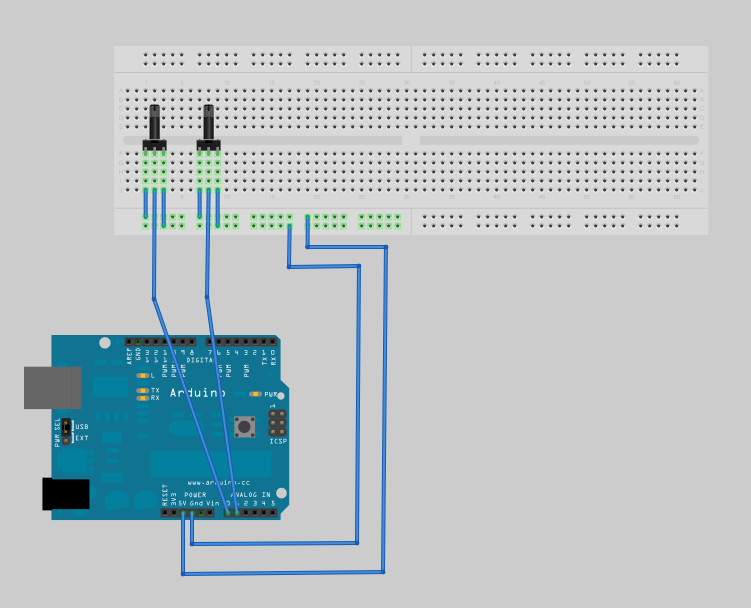— craig_fahner @ 4:16 amArduino Code:

```int sensorValue0;
int sensorValue1;

void setup() {
// initialize the serial communication:
Serial.begin(9600);
}

void loop() {
Serial.print(sensorValue0);
Serial.print(",");
Serial.println(sensorValue1);

delay(10);
}```

Processing code:

```import processing.serial.*;

int sensor0 = 0;
int sensor1 = 0;

Serial myPort;

void setup() {
size(200, 200);

// List all the available serial ports
println(Serial.list());
// I know that the first port in the serial list on my mac
// is always my  Arduino, so I open Serial.list().
// Open whatever port is the one you're using.
myPort = new Serial(this, Serial.list(), 9600);
// don't generate a serialEvent() unless you get a newline character:
myPort.bufferUntil('\n');
}

void draw() {
}

void serialEvent(Serial myPort) {
// get the ASCII string:

if (inString != null) {
// trim off any whitespace:
inString = trim(inString);
// split the string on the commas and convert the
// resulting substrings into an integer array:
int[] sensors = int(split(inString, ","));
// if the array has at least two elements, you know
// you got the whole thing.  Put the numbers in the
// sensor variables:
if (sensors.length >=2) {
sensor0 = sensors;
sensor1 = sensors;
}
}
}```